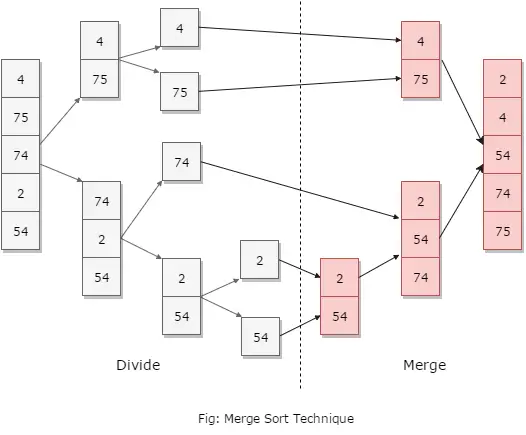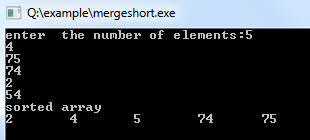# Merge Sort Algorithm

Merge sort is another sorting technique and has an algorithm that has a reasonably proficient space-time complexity - O(n log n) and is quite trivial to apply. This algorithm is based on splitting a list, into two comparable sized lists, i.e., left and right and then sorting each list and then merging the two sorted lists back together as one.

Merge sort can be done in two types both having similar logic and way of implementation. These are:

• Top down implementation
• Bottom up implementation

Below given figure shows how Merge Sort works:### Algorithm for Merge Sort

1. algorithm Merge_Sort(list)
2. Pre: list 6= fi
3. Post: list has been sorted into values of ascending order
4. if list.Count = 1 // when already sorted
5. return list
6. end if
7. m <- list.Count = 2
8. left <- list(m)
9. right <- list(list.Count - m)
10. for i <- 0 to left.Count - 1
11. left[i] <- list[i]
12. end for
13. for i <- 0 to right.Count -1
14. right[i] <- list[i]
15. end for
16. left <- Merg_Sort(left)
17. right <- Merge_Sort(right)
18. return MergeOrdered(left, right)
19. end Merge_Sort
It is to be noted that, Merge sort uses the concept of divide and conquer technique that was invented by John von Neumann in the year 1945.

Example:

``````#include <iostream>
using namespace std;

void merge(int *,int, int , int );
void mergesort(int *a, int low, int high)
{
int mid;
if (low < high)
{
mid=(low+high)/2;
mergesort(a,low,mid);
mergesort(a,mid+1,high);
merge(a,low,high,mid);
}
return;
}
// Merge sort concepts starts here
void merge(int *a, int low, int high, int mid)
{
int i, j, k, c;
i = low;
k = low;
j = mid + 1;
while (i <= mid && j <= high)
{
if (a[i] < a[j])
{
c[k] = a[i];
k++;
i++;
}
else
{
c[k] = a[j];
k++;
j++;
}
}
while (i <= mid)
{
c[k] = a[i];
k++;
i++;
}
while (j <= high)
{
c[k] = a[j];
k++;
j++;
}
for (i = low; i < k; i++)
{
a[i] = c[i];
}
}
// from main mergesort function gets called
int main()
{
int a, i, b;
cout<<"enter  the number of elements:";
for (i = 0; i <= 5; i++) { cin>>a[i];
}
mergesort(a, 0, 4);
cout<<"sorted array\n";
for (i = 0; i < 5; i++)
{
cout<<a[i]<<"\t";
}
cout<<"enter  the number of elements:";
for (i = 0; i < 5; i++) { cin>>b[i];
}
mergesort(b, 0, 4);
cout<<"sorted array:\n";
for (i = 0; i < 5; i++)
{
cout<<b[i]<<"\t";
}
getch();
}``````

Output: